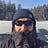## Thomas HuaultThomas Huault

8 months ago

## DATA MINING WITH SUPERALGORES

Old pots produce the best soup.Science has always inspired indicator design. From physics to signal processing, many indicators use concepts from mechanical engineering, electronics, and probability. In Superalgos' Data Mining section, we've explored using thermodynamics and information theory to construct indicators and using statistical and probabilistic techniques like reduced normal law to take advantage of low probability events.

An asset's price is like a mechanical object revolving around its moving average. Using this approach, we could design an indicator using the oscillator's Total Energy. An oscillator's energy is finite and constant. Since we don't expect the price to follow the harmonic oscillator, this energy should deviate from the perfect situation, and the maximum of divergence may provide us valuable information on the price's moving average.

# Definition of the Harmonic Oscillator in Few Words

Sinusoidal function describes a harmonic oscillator. The time-constant energy equation for a harmonic oscillator is: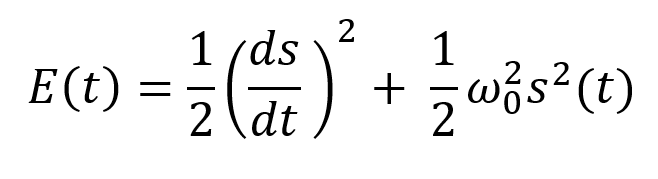With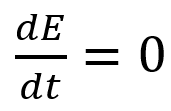Time saves energy.

In a mechanical harmonic oscillator, total energy equals kinetic energy plus potential energy. The formula for energy is the same for every kind of harmonic oscillator; only the terms of total energy must be adapted to fit the relevant units. Each oscillator has a velocity component (kinetic energy) and a position to equilibrium component (potential energy).

# The Price Oscillator and the Energy Formula

Considering the harmonic oscillator definition, we must specify kinetic and potential components for our price oscillator. We define oscillator velocity as the rate of change and equilibrium position as the price's distance from its moving average.

Price kinetic energy: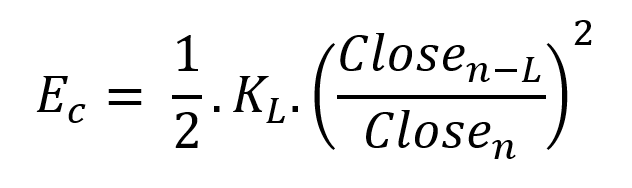It's like: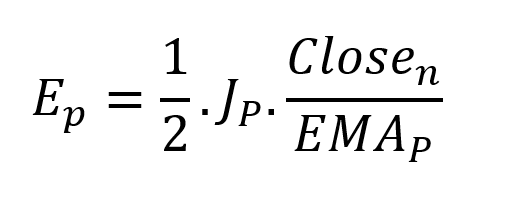With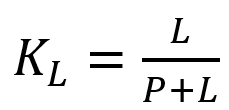and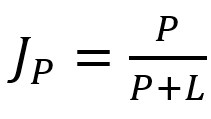L is the number of periods for the rate of change calculation and P for the close price EMA calculation.

Total price oscillator energy =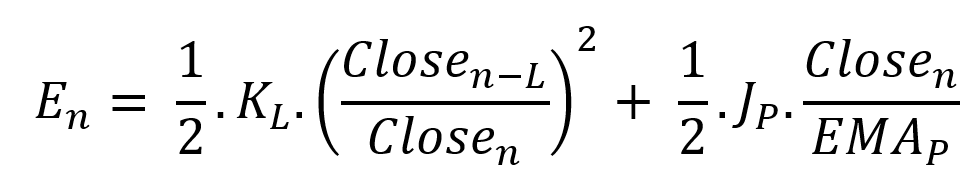Given that an asset's price can theoretically vary at a limitless speed and be endlessly far from its moving average, we don't expect this formula's outcome to be constrained. We'll normalize it using Z-Score for convenience of usage and readability, which also allows probabilistic interpretation.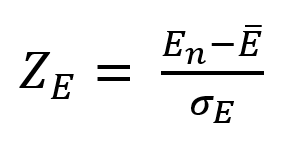Over 20 periods, we'll calculate E's moving average and standard deviation.

We calculated Z on BTC/USDT with L = 10 and P = 21 using Knime Analytics.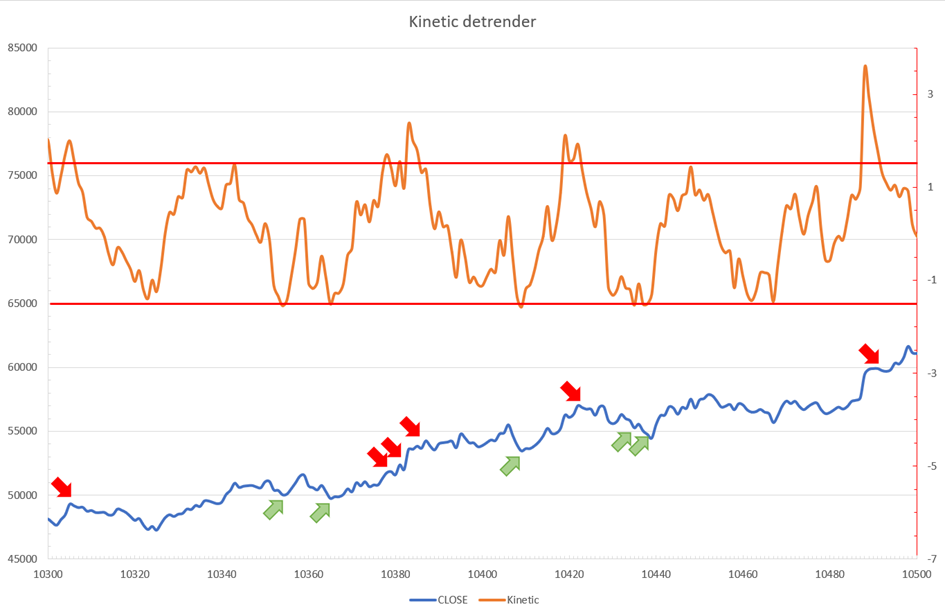The graph is detrended. We added two horizontal lines at +/- 1.6 to construct a 94.5% probability zone based on reduced normal law tables. Price cycles to its moving average oscillate clearly. Red and green arrows illustrate where the oscillator crosses the top and lower limits, corresponding to the maximum/minimum price oscillation. Since the results seem noisy, we may apply a non-lagging low-pass or multipole filter like Butterworth or Laguerre filters and employ dynamic bands at a multiple of Z's standard deviation instead of fixed levels.

# Kinetic Detrender Implementation in Superalgos

The Superalgos Kinetic detrender features fixed upper and lower levels and dynamic volatility bands.

The code is pretty basic and does not require a huge amount of code lines.

It starts with the standard definitions of the candle pointer and the constant declaration :

``````let candle = record.current
let len = 10
let P = 21
let T = 20
let up = 1.6
let low = 1.6``````

Upper and lower dynamic volatility band constants are up and low.

We proceed to the initialization of the previous value for EMA :

``````if (variable.prevEMA === undefined) {
variable.prevEMA = candle.close
}``````

And the calculation of EMA with a function (it is worth noticing the function is declared at the end of the code snippet in Superalgos) :

``````variable.ema = calculateEMA(P, candle.close, variable.prevEMA)
//EMA calculation
function calculateEMA(periods, price, previousEMA) {
let k = 2 / (periods + 1)
return price * k + previousEMA * (1 - k)
}``````

The rate of change is calculated by first storing the right amount of close price values and proceeding to the calculation by dividing the current close price by the first member of the close price array:

``````variable.allClose.push(candle.close)
if (variable.allClose.length > len) {
variable.allClose.splice(0, 1)
}
if (variable.allClose.length === len) {
variable.roc = candle.close / variable.allClose
} else {
variable.roc = 1
}``````

Finally, we get energy with a single line:

``variable.E = 1 / 2 * len * variable.roc + 1 / 2 * P * candle.close / variable.ema``

The Z calculation reuses code from Z-Normalization-based indicators:

``````variable.allE.push(variable.E)
if (variable.allE.length > T) {
variable.allE.splice(0, 1)
}
variable.sum = 0
variable.SQ = 0
if (variable.allE.length === T) {
for (var i = 0; i < T; i++) {
variable.sum += variable.allE[i]
}
variable.MA = variable.sum / T
for (var i = 0; i < T; i++) {
variable.SQ += Math.pow(variable.allE[i] - variable.MA, 2)
}
variable.sigma = Math.sqrt(variable.SQ / T)
variable.Z = (variable.E - variable.MA) / variable.sigma
} else {
variable.Z = 0
}
variable.allZ.push(variable.Z)
if (variable.allZ.length > T) {
variable.allZ.splice(0, 1)
}
variable.sum = 0
variable.SQ = 0
if (variable.allZ.length === T) {
for (var i = 0; i < T; i++) {
variable.sum += variable.allZ[i]
}
variable.MAZ = variable.sum / T
for (var i = 0; i < T; i++) {
variable.SQ += Math.pow(variable.allZ[i] - variable.MAZ, 2)
}
variable.sigZ = Math.sqrt(variable.SQ / T)
} else {
variable.MAZ = variable.Z
variable.sigZ = variable.MAZ * 0.02
}
variable.upper = variable.MAZ + up * variable.sigZ
variable.lower = variable.MAZ - low * variable.sigZ``````

We also update the EMA value.

``variable.prevEMA = variable.EMA``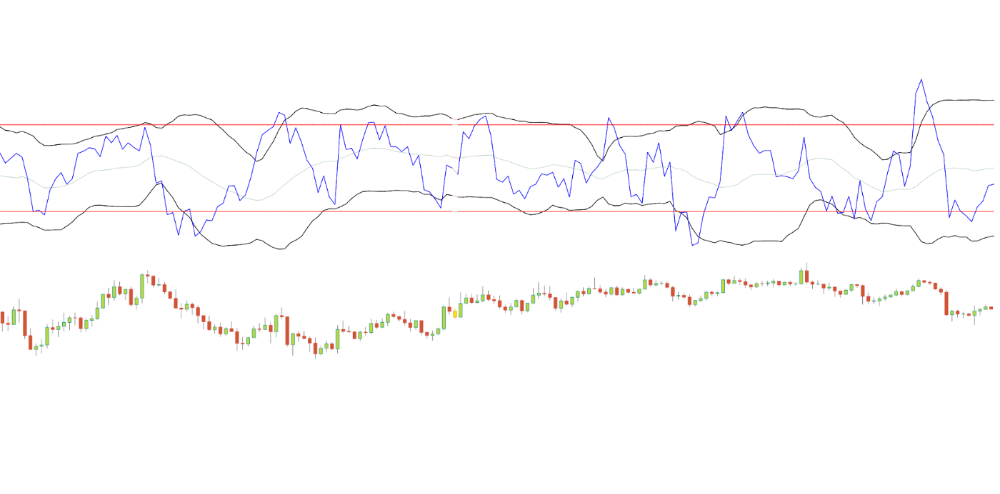# Conclusion

We showed how to build a detrended oscillator using simple harmonic oscillator theory. Kinetic detrender's main line oscillates between 2 fixed levels framing 95% of the values and 2 dynamic levels, leading to auto-adaptive mean reversion zones.

Superalgos' Normalized Momentum data mine has the Kinetic detrender indication.

All the material here can be reused and integrated freely by linking to this article and Superalgos.

This post is informative and not financial advice. Seek expert counsel before trading. Risk using this material.